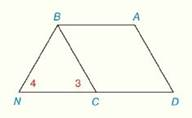Chapter 4.CR, Problem 29CR### Elementary Geometry for College St...

6th Edition
Daniel C. Alexander + 1 other
ISBN: 9781285195698

#### Solutions

Chapter
Section### Elementary Geometry for College St...

6th Edition
Daniel C. Alexander + 1 other
ISBN: 9781285195698
Textbook Problem
47 views

# Review Exercises Given: A B C D is a parallelogram D C - ≅ B N - ∠ 3 ≅ ∠ 4 Prove: A B C D is a rhombusTo determine

To Prove:

The provided quadrilateral ABCD is a rhombus.

Explanation

Proof:

Definition:

A rhombus is a parallelogram with two congruent adjacent sides.

Theorem on parallelogram:

The opposite sides of a parallelogram are congruent.

Description:

It is given that ABCD is a parallelogram with DC¯BN¯ and 34.

Since 34, BCN is an isosceles triangle with BN=BC

### Still sussing out bartleby?

Check out a sample textbook solution.

See a sample solution

#### The Solution to Your Study Problems

Bartleby provides explanations to thousands of textbook problems written by our experts, many with advanced degrees!

Get Started